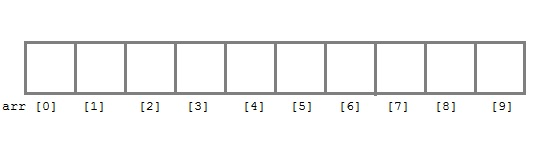# Introduction to Array

Array in C is s type of data structure that can store similar type of data in continuous blocks of memory.
For example, an array of integer can only store integer type values and an array of float type can only store float type values.## Declaring an array Syntax:

`data type array_name [array size];`
• Data type – data type will tell about which type of a value array will accept.
For example: int ar [ ], float bk [ ] etc. In the above example ar is the integer type of array and bk is the float type of array.
• Array name – An array has a specific name that you can give except special characters and reserved words.
• Array size – Array size tells about the size of the array that how many variables it can store. VERY
• IMPORTANT: Array indices start at zero in C, and go to one less than the size of the array.  For example, a five element array will have indices zero through four.  This is because the index in C is actually an offset from the beginning of the array.  ( The first element is at the beginning of the array, and hence has zero offset. )

## Initializing an array

1. Compile time initialization
There are multiple ways to initialize an array.
a) . Example

`float cap = {9,8,7,6,5,4};`

You can initialize the array at the declaration time only.
int max = {1,2,3,4,5,}; // one important thing to remember is that when you will give more initializer (array elements) than declared array size than the compiler will give an error.

b) . Example

`int cap[ ] = {2,3,4,5,6,7,8,9,0,3};`

We don’t have to mention the size of the array, in this case as we have defined the elements in the curly braces so the compiler will know.
And there are many other approaches that we will be covered in advance course.

// Program of compile time initialization

 Declaration/Initialization of Array Examples Array declaration syntax: data_type arr_name [arr_size]; int age; Array initialization syntax: data_type arr_name[arr_size] = {value1, value2,....valueN}; int age={1,2,3,4,5}; Array accessing syntax: array_name[index]; age; /* 1 */ age; /* 2 */

2. Run time initialization
An array can also be initialized at runtime using scanf() function. This approach is usually used for initializing large array, or to initialize array with user specified values.

Example,
//program of initializing at runtime